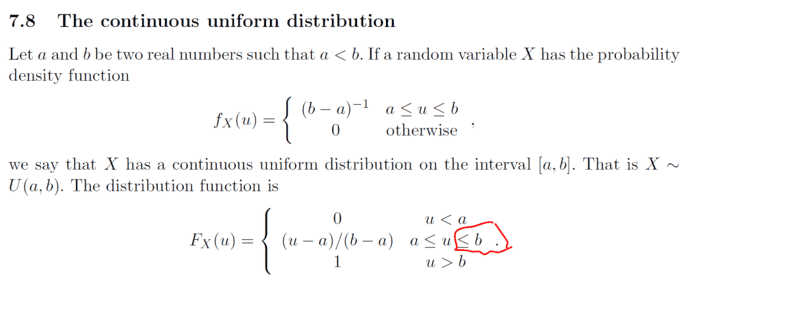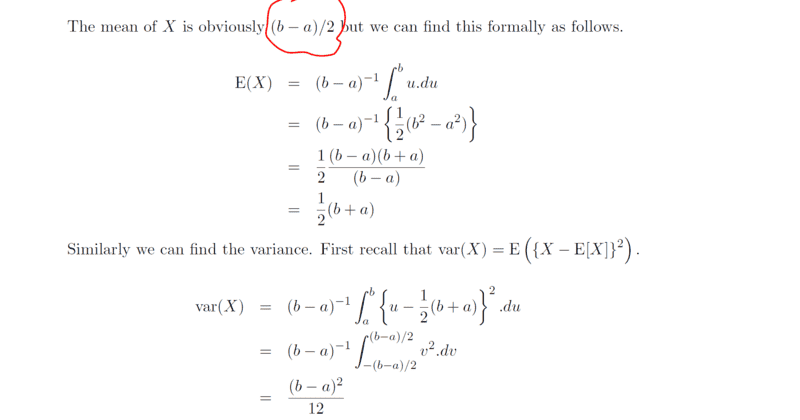# Understanding the concept of Probability distribution

• chwala
In summary, the problem appears to be that when solving for ##F_X(u)## using the given probability density function, the value of ##F_X(u)## is equal to ##1## when ##u=b##. This is a mistake because the correct value of ##F_X(u)## is ##\dfrac{1}{b-a}##.

#### chwala

Gold Member
Homework Statement
see attached
Relevant Equations
statistics
Consider the attachment below;How did they arrive at

##F_X (u) = \dfrac{u-a}{b-a}## ?

I think there is a mistake on the inequality, probably its supposed to be ##a≤u<b## and that will mean;

$$F_X (u) =\dfrac{1}{b-a} \int_a^u du= \dfrac{1}{b-a} ⋅(u-a)$$ as required. Your thoughts...then i have the second part of the question that i will post here after the analysis.

Last edited:
What do you think goes wrong if you include b? What is ##F(b)## supposed to be, and what does it become?

Office_Shredder said:
What do you think goes wrong if you include b?
It will be equal to ##1##.

chwala said:
It will be equal to ##1##.
And why would that be a problem?

Do note that the definition of ##F_X## would result in exactly the same function if you instead defined the middle case on the interval ##[a,b)## and the third on ##[b, \infty)## because both expressions are equal to one at ##u = b##.

Orodruin said:
And why would that be a problem?

Do note that the definition of ##F_X## would result in exactly the same function if you instead defined the middle case on the interval ##[a,b)## and the third on ##[b, \infty)## because both expressions are equal to one at ##u = b##.
Ok, i hear you...then are my steps in post ##1## correct with the given limits? if not then how did they arrive at the solution.

Orodruin said:
And why would that be a problem?

Do note that the definition of ##F_X## would result in exactly the same function if you instead defined the middle case on the interval ##[a,b)## and the third on ##[b, \infty)## because both expressions are equal to one at ##u = b##.

My intention is to get the shown ##F_X(u)## from the given probability density function ##f_x(u)=(b-a)^{-1}##. This is where my problem is.This is the continuation of the same problem...clearly textbook mistake on ##E(x)## value i.e the highlighted in red...

Anyway, my approach on this problem;

$$E(x)=\dfrac{1}{b-a} \int_a^b u du= \left[\dfrac{b^2-a^2}{2} ⋅\dfrac{1}{b-a}\right]=\left[\dfrac{(b+a)(b-a)}{2(b-a)}\right]=\dfrac{b+a}{2}$$

$$Var(x)=\dfrac{1}{b-a} \int_a^b u^2 du=\left[\dfrac{b^3-a^3}{3} ⋅\dfrac{1}{b-a}\right]=\left[\dfrac{b^2+a^2+ab}{3}-\dfrac{(b+a)^2}{4}\right]$$

$$=\left[\dfrac{(4b^2+4a^2+4ab)-(3b^2+6ab+3a^2)}{12}\right]$$

$$=\left[\dfrac{b^2+a^2-2ab}{12}\right]= \dfrac{(b-a)^2}{12}$$

if there is a better approach will appreciate. Cheers guys!

Last edited: